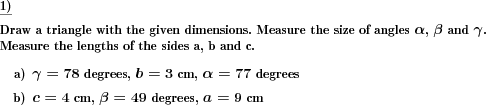Custom math worksheets at your fingertipsDetails for problem "Draw a triangle with given dimensions"

Quickname: 3913

Junior High School, Middle School, High School.

Summary

Dimensions are given that define triangle. The corresponding triangle has to be drawn and sides and/or angles measured.

ExampleDescription

Some dimensions of a triangle are given. The corresponding triangle has to be drawn.

The dimensions given can be chosen to be either

• the lengths of the three sides
• the size of two angles and the length of the common side
• the length of two sides and the size of the angle between these sides
• or a random selection of the three possibilities above.

Optionally, the length of the sides and/or size of the angles can be asked for. These have to be determined by measurement.

Download free printable worksheets for this math problem here. The worksheet contains the problems only, the solution sheet includes the answers. Just click on the respective link.

•Worksheet 1Solution sheet with answers
•Worksheet 2Solution sheet with answers
•Worksheet 3Solution sheet with answers

If you can not see the solution sheets for download, they may be filtered out by an ad blocker that you may have installed. If this is the case, please allow ads for this page and reload the page. The solution sheets will then reappear.

These worksheets contain math problems that have to be printed with the correct aspect ratio. When printing, please take care to switch all automatic adjustments, scaling of page, auto adjust, etc, off.

• Do these sample worksheets do not really fit?
• Do you need more math worksheets, with a different level of difficulty?
• Would you like to combine different problems on a worksheet and adjust them to your needs?
• As a teacher, you can put together your own worksheets using the automatically generated math problems provided.
With a free initial credit, you can start creating your own math worksheets in a few minutes.

You can try it for free! Register here, to create custom worksheets now!

Customization options for this problem

Parameter
Possible values
Number of problems
1, 2, 3, 4
Given dimenstions
sides, angle,side,angle, side,angle,side, sas or asa, sss or sas, asa or sas, sss, sas or asa
Measure angles
Yes, No
Measure sides
Yes, NoDeutsche Version dieser Aufgabe
These informational pages with samples describe math problems that can be combined on custom math worksheets with solutions for home and K-12 school use.
Deutsche Seiten
×